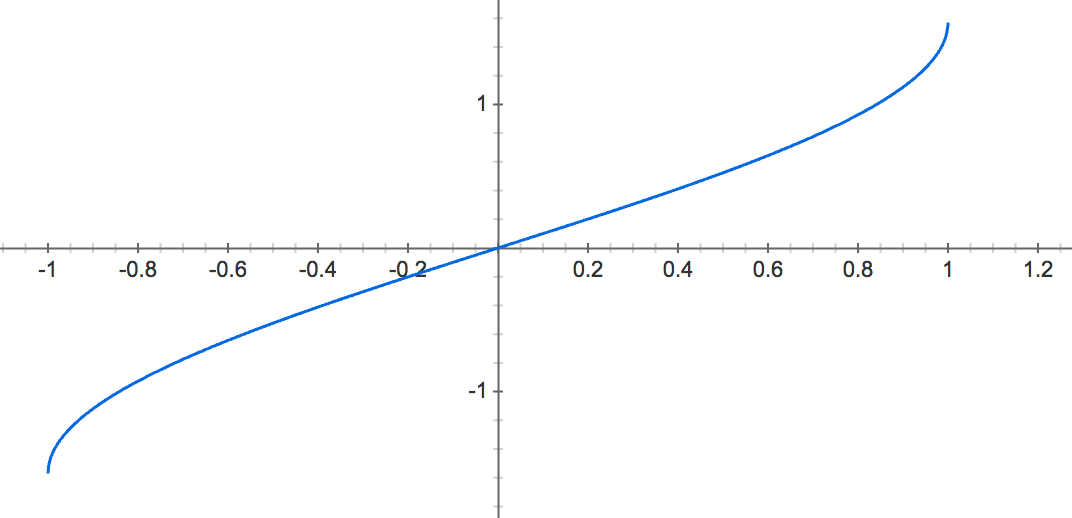# Arcsin(x) function

arcsin(x), sin-1(x), inverse sine function.

Contents

### Arcsin definition

The arcsine of x is defined as the inverse sine function of x when -1≤x≤1.

When the sine of y is equal to x:

sin y = x

Then the arcsine of x is equal to the inverse sine function of x, which is equal to y:

arcsin x = sin-1 x = y

For example:

arcsin 1 = sin-1 1 = π/2 rad = 90°

### Graph of arcsin, f(x) = asin(x)